Why should we always divide notes in two? Why would one not be able to divide it in 3 or 5? Let's mess with the rules ;)

During the previous weeks, I have been working on tuplets notation for Flat.io. In this post, I am going to describe the logic behind it.

Tuplets are used to express an irregular rhythm. You can for instance divide a note in three where it would normally be divided in two, or the opposite.

Tuplets are defined with a ratio. The ratio between the number of normal notes,
and the number of notes actually in the tuplet.

Normal notes are the notes that would be played if there were no tuplet. Actual notes are the notes actually played with the tuplet.

### Simple examples

#### Triplet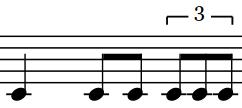A triplet is a tuplet with a ratio of 3 notes actually played in the place of 2 normal notes. The ratio is 3:2.

#### Quintuplet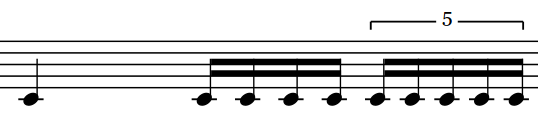A quintuplet is a tuplet with a ratio of 5 notes actually played in the place of 4 normal notes. The ratio is 5:4.

#### Sextuplet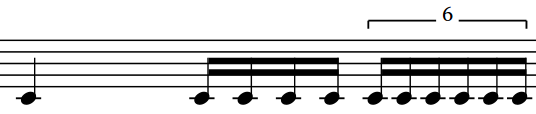A sextuplet is a tuplet with a ratio of 6 notes actually played in the place of 4 normal notes. The ratio is 6:4
Note that a sextuplet is equivalent to two triplets: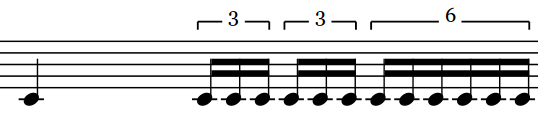### Multiple durations

Sometimes the notes of a tuplet do not have the same duration. You can have for instance a triplet, with one quarter note and two sixteenth. For the convenience, we use internally an other value. This is the duration that each note of the tuplet would have if they had the same duration. For instance, with this tuplet, we state that the tuplet note duration is 8th.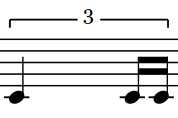### Use cases

It is possible to have groups of three notes with a time signature like 12/8.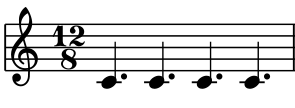You can also achieve this result with a time signature of 4/4 and triplets all along.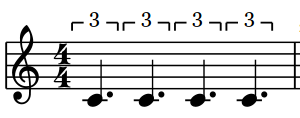It can also be interesting to mix tuplets with normal time, or either with other tuplet types, for instance to create interesting rhythms for drummers for instance.

### Tuplets with Flat

Here are the tuplet types supported by Flat.io.

• 3/2
• 5/4
• 6/4

You can only start a tuplet from a note without dots. This is because is has to be a note that can be divided in a number of shards that is a power of two. If it were a dotted note, the first level of divisions would be three.

That's all for this first presentation. The internal code also supports septuplets, nontuplets, duplets and quadruplets, but we have to figure out how to integrate it without cluttering the user interface.
I will write another article about tuplets when I will be working on nested tuplets.
Take care of yourself and enjoy awesome compositions with this new notation!